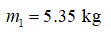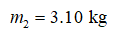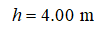# Two objects(m1 = 5.35 kgandm2 = 3.10 kg)are connected by a light string passing over a light, frictionless pulley as in the figure below. The 5.35-kg object is released from rest at a pointh = 4.00 mabove the table.Two objects are connected by a taught string passing over a pulley. An object labeled m2 rests on a table, and an object labeled m1 dangles a distance h above the table.(a) Determine the speed of each object when the two pass each other.m/s(b) Determine the speed of each object at the moment the 5.35-kg object hits the table.m/s(c) How much higher does the 3.10-kg object travel after the 5.35-kg object hits the table?

Question
1 views

Two objects

(m1 = 5.35 kg

and

m2 = 3.10 kg)

are connected by a light string passing over a light, frictionless pulley as in the figure below. The 5.35-kg object is released from rest at a point

h = 4.00 m

above the table.

Two objects are connected by a taught string passing over a pulley. An object labeled m2 rests on a table, and an object labeled m1 dangles a distance h above the table.
(a) Determine the speed of each object when the two pass each other.
m/s

(b) Determine the speed of each object at the moment the 5.35-kg object hits the table.
m/s

(c) How much higher does the 3.10-kg object travel after the 5.35-kg object hits the table?
check_circle

Step 1

Mass of the object 1,Step 2

Mass of the object 2,Step 3

The initial height of object 1,...

### Want to see the full answer?

See Solution

#### Want to see this answer and more?

Solutions are written by subject experts who are available 24/7. Questions are typically answered within 1 hour.*

See Solution
*Response times may vary by subject and question.
Tagged in